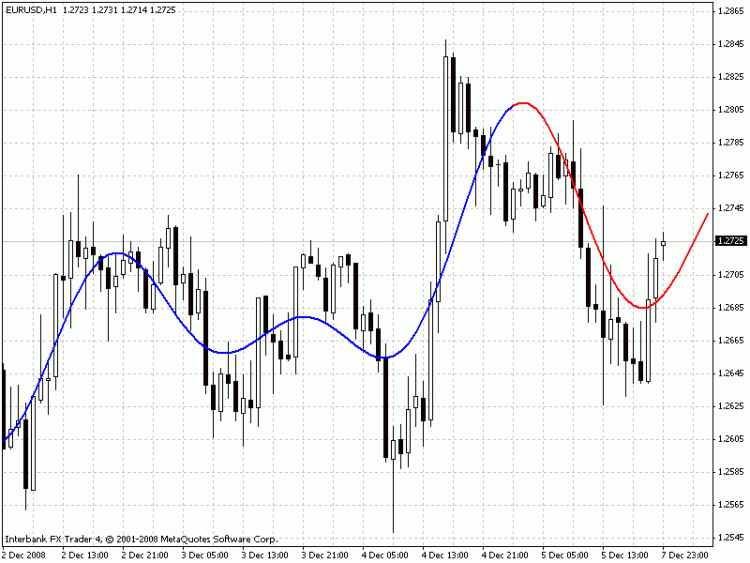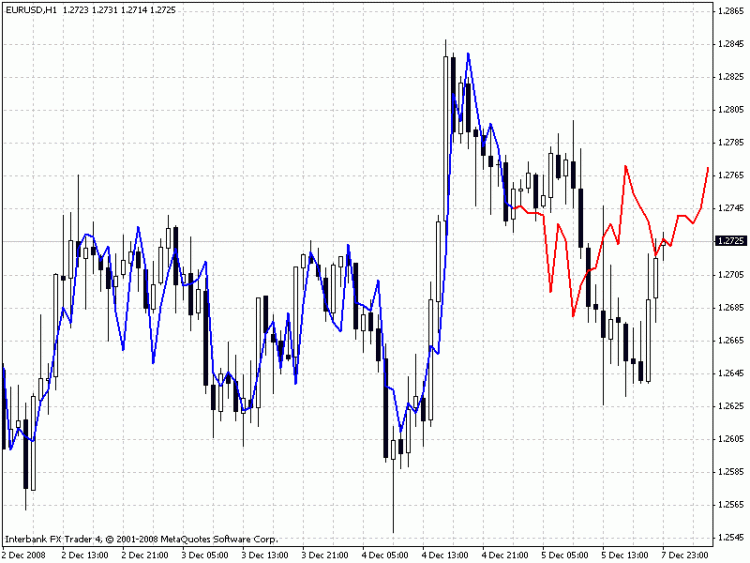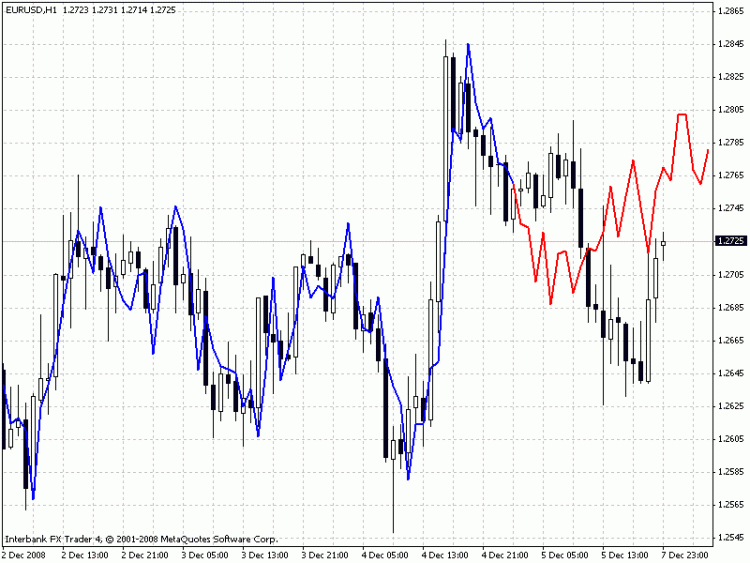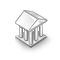Interesting script?
So post a link to it -
let others appraise it
You liked the script? Try it in the MetaTrader 5 terminal# Extrapolator - indicator for MetaTrader 4

Views:
55115
Rating:
Published:
2008.12.25 07:18
Updated:
2016.11.22 07:32

The indicator is based on several methods that can be chosen by the Method input variable:

Method 1: Fourier's extrapolation; the frequencies are calculated using the Quinn-Fernandes Algorithm

Method 2: Autocorrelation Method

Method 3: Weighted Burg Method

Method 4: Burg Method with Helme-Nikias weighting function

Method 5: Itakura-Saito (geometric) method

Method 6: Modified covariance method

Methods 2-6 are the methods of linear prediction. The linear prediction is based on finding the future values as the linear functions of the past values. Assume that we have a number of prices x..x[n-1] where the higher index is compliant with the recent price. The prediction of the future price x[n] is calculated as

x[n] = -Sum(a[i]*x[n-i], i=1..p)

where a[i=1..p] - coefficients of the model, p - order of the model. The listed methods 2-6 find the coefficients a[] by decreasing the mean-root-square error on the training last n-p bars. Of course, we can reach the zero error of prediction if we directly solve the set of equations mentioned above with n=2*p by the Levinson-Durbin method. Such method of prediction is called Prony Method. Its disadvantage is the instability during the prediction of the future values of the series. That's why this method has not been included.

The other input parameters are:

LastBar - the number of the last bar in the past data

PastBars - the number of past bars used for the prediction of the future values

LPOrder - the order of the linear model as a fraction from the number of the past bars (0..1)

FutBars - the number of future bars in the prediction

HarmNo - the maximum number of frequencies for the Method 1 (0 means all frequencies)

FreqTOL - the imprecision of the frequeincies calculation for the Method 1 (if it is >0.001 it can't converge)

BurgWin - the number of the weighting function for the Method 2 (0=Rectangular 1=Hamming 2=Parabolic)

The indicator draws two lines: the blue line shows the prices of the model on the training bars, the red line shows the predicted future prices.

Examples:

Method 1 (the extrapolation of Fourier series)Method 3 (Burg's method)Method 6 (Modified Covariance Method)Petition:

If somebody will be successful in developing a profitable EA based on this indicator I ask to share his/her ideas via the e-mail specified inside the code.

Translated from Russian by MetaQuotes Software Corp.
Original code: https://www.mql5.com/ru/code/8608Burg Extrapolator

The EA uses the method of Burg's linear prediction that was taken from my indicator Extrapolator.mq4.Ryan_Jones_SM

It shows where to buy and where to sell via the arrows. If a trend occurs then it will bring profit, if there is no trend, there will be no profit.Capture MouseEvents on Chart

This set of functions enables one to capture mouse events on your trading chart. One can simmulate buttons to trigger actions in the EA.Volatility Indicator

Judging by the code of indicator, it calculates the difference between the maximum maximums and minimum minimums of prices of the candlesticks for the last 20 bars (parameter). The result is displayed in points.